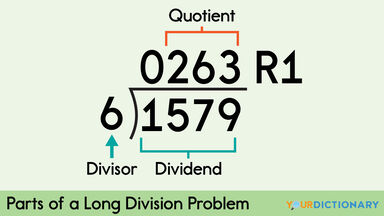# Steps to Long Division Problems (With Examples)

Math can be tricky. Make it simple by breaking a difficult topic like division into easy-to-follow long division steps. That's just what long division is. It’s a way to break the division of large numbers into simple steps. Learn the steps through several long division examples.parts of long division problem

## What Is Long Division?

Dividing 543,439 by 31 would be challenging to do in your head. So, rather than focusing all your brainpower on division, you can use a long division method, which breaks the numbers into steps. These steps allow you to take one part of the number at a time, making the math so easy even a fourth grader can do it.

### Terms Used in Long Division

Before you learn how to complete long division, it’s essential to get familiar with a few vital math terms. You may see a division problem written in different ways, such as using a ÷ or a / to indicate "divided by."

• dividend - the number that needs dividing
• divisor - the number you are dividing by
• remainder - the leftover amount when dividend doesn't divide equally

Therefore, in the equation 1327 / 25 = 53 R2, 1327 is the dividend, 25 is the divisor, 53 is the quotient, and 2 is the remainder. Alright, now that you’ve got the basics, it’s time to dive right into how to divide.

## Simple Long Division Steps

Typically, long division is broken down into five different steps. Explore each separate step using the equation:

1579 / 6 = x.

### Step 1: Divide

Long division is all about breaking an equation into different parts. Therefore, rather than looking at the whole equation, you look at the first number of the dividend, which in the equation 1579 / 6 is the number 1. Ask yourself: how many 6s are in 1? Since 1 is less than 6, your answer would be 0.

### Step 2: Multiply

Now that you know that 6 will go into 1 zero times, then you need to multiply (6 * 0 = 0). Place the zero under the 1 in the equation.

### Step 3: Subtract

Now it is time for you to subtract the numbers from one another (1 - 0 =1). You will write the difference under the line in your equation.

### Step 4: Bring the Number Down

Once the subtraction is done, you bring the next number in the equation down. In our dividend, you would need to bring the 5 down.

### Step 5: Repeat

Once you understand steps 1-4, you just need to repeat the division, multiplication, subtraction, and bringing the number down until there aren’t any more numbers for your to bring down. So for our equation, you find out that 1579 / 6 = 263 R1.

## Long Division Examples

Now that you know how to do a long division problem, it's time to try a few examples yourself. You'll need to write these down using the standard formatting for a division problem.

• 1204 / 4
4 goes into 1 zero times
1 - 0 = 1, 2 drops down
4 goes into 12 three times (first number in the answer is 3)
12 - 12 = 0, 0 drops down
4 goes into 0 zero times (second number in the answer is 0)
0 - 0 = 0, 4 drops down
4 goes into 4 one time (third number in the answer is 1), answer is 301
• 3024 / 24
24 goes into 3 zero times
3 - 0 = 3, 0 drops down
24 goes into 30 one time (first number in the answer is 1)
30 - 24 = 6, 2 drops down to make 62
24 goes into 62 two times (second number in the answer is 2)
62 - 48 = 14, 4 drops down to make 144
24 goes into 144 six times (last number is 6), answer is 126
• 675 / 5
5 goes into 6 one time (first number in the answer is 1)
6 - 5 = 1, 7 drops down
5 goes into 17 three times (second number in the answer is 3)
17 - 15 = 2, 5 drops down
5 goes into 25 five times (last number in the answer is 5), answer is 135
• 679 / 5
5 goes into 6 one time (first number in the answer is 1)
6 - 5 = 1, 7 drops down
5 goes into 17 three times (second number in the answer is 3)
17 - 15 = 2, 9 drops down
5 goes into 29 five times (last number in the answer is 5)
you have a remainder of 4, answer is 135 R4
Check your work (5 * 135) + 4 = 679

## Why Do Some Equations Have a Remainder

Not every divisor you have in long division will divide equally. Therefore, most of the time, you have a leftover bit that can’t be divided by the number any further. This is called your remainder. It’s represented in a math equation with an R. Once you advance a bit further into rational numbers, you’ll see how the remainder fits in!